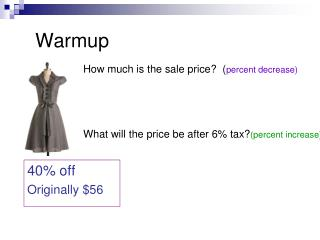DownloadDownload PresentationWarmup

# Warmup

Download Presentation## Warmup

- - - - - - - - - - - - - - - - - - - - - - - - - - - E N D - - - - - - - - - - - - - - - - - - - - - - - - - - -
##### Presentation Transcript

1. Warmup How much is the sale price? (percent decrease) What will the price be after 6% tax?(percent increase) 40% off Originally \$56

2. Mixture Problems Objective: To solve mixture problems. Standard 5.0

3. Mixture Notes Paper 1) Les spent \$4.40 for several pencils costing 10 cents each and some notebooks costing 80 cents each. He bought 8 more pencils than notebooks. How many notebooks did he buy? Quantity ∙ cost = total value Multiply across Add down Pencils Notebooks Mixture 10(x + 8) + 80(x) = 440 10x + 80 + 80x = 440 90x + 80 = 440 90x = 360 x = 4 notebooks so 12 pencils

4. Mixture Notes Paper 2) Susan makes \$3 an hour working after school and \$4 an hour working on Saturdays. Last week she worked a total of 12 hours and made \$42.50. How many hours did she work on Saturday? hours ∙ rate = total value Multiply across Add down school Saturdays Mixture 3(12 – x) + 4x = 42.50 36 – 3x + 4x = 42.50 36 + x = 42.50 x = 6.50 6 hours 30 min on Saturdays

5. Mixture Notes Paper 3) Raisins are worth \$3.24 per pound was mixed with dried apricots worth \$4.22 per pound to produce a mixture worth \$3.80 per pound. If there were 15 pounds of raisins then calculate the amount of dried apricots in the mixture. Quantity ∙ cost = total value Multiply across Add down Raisins Apricots Mixture 15(324) + x(422) = 380(15 + x) 4860 + 422x = 5700 + 380x 4860 + 42x = 5700 42x = 840 x = 20 apricots

6. Homework • Plowed Field Show all of your work on a separate piece of paper to receive full credit!